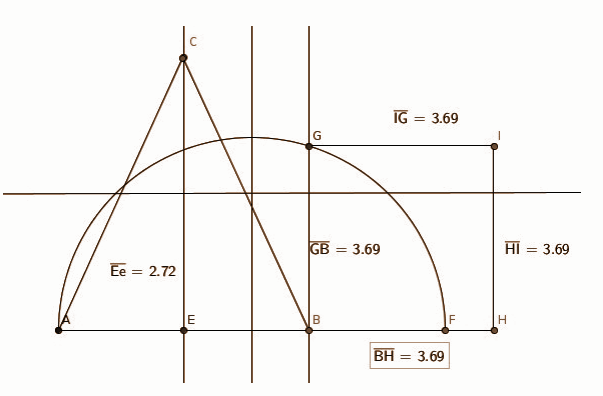# Kerala SSLC Board Question Papers for Class 10th Maths 2015 With Solutions In PDF

Kerala SSLC (Class 10) maths 2015 question paper with solutions are provided here in a downloadable pdf format and also in the text so that the students can easily access them. Along with the solutions, they can also get the maths question paper 2015 Class 10 SSLC for reference. Students are able to access all the Kerala board previous year maths question papers here. These can be downloaded easily and students can practice and verify the answers provided by BYJU’S. Solving 2015 Maths question papers for class 10 will help the students to predict what type of questions will appear in the exam.

### QUESTION PAPER CODE S 127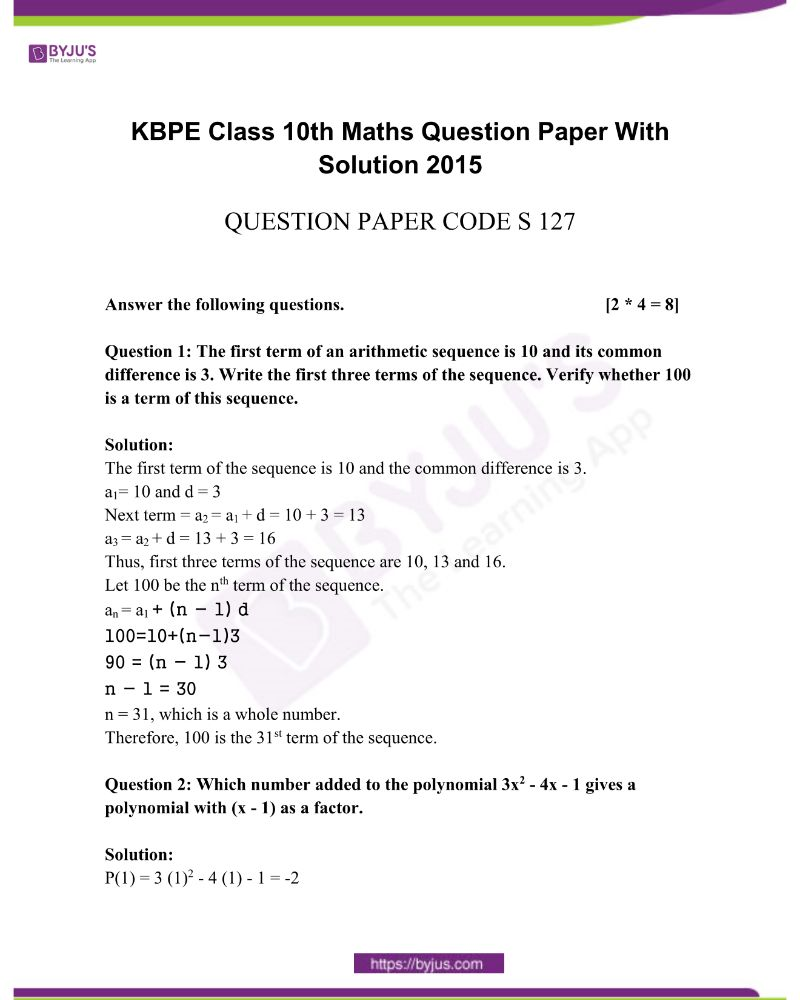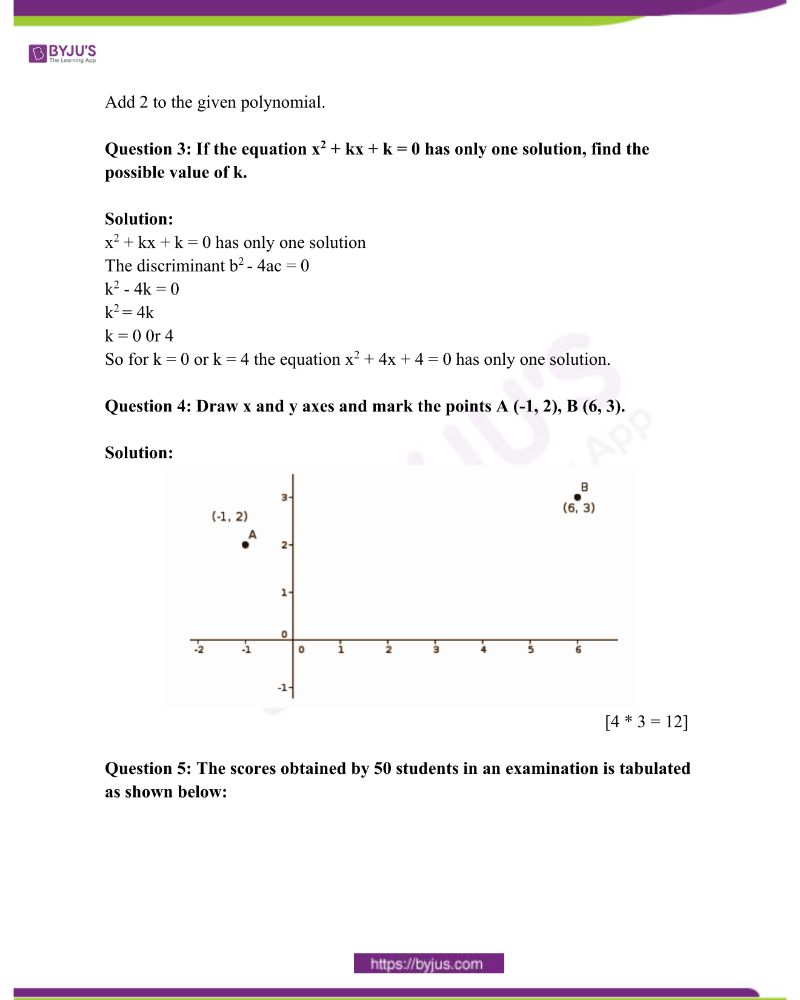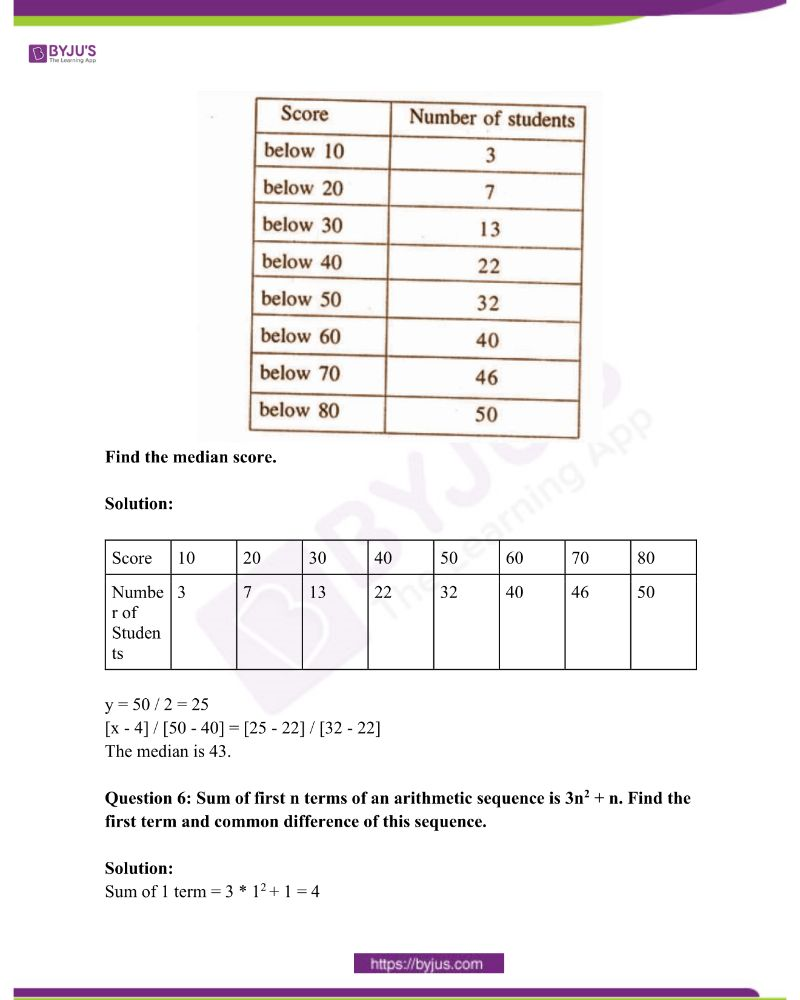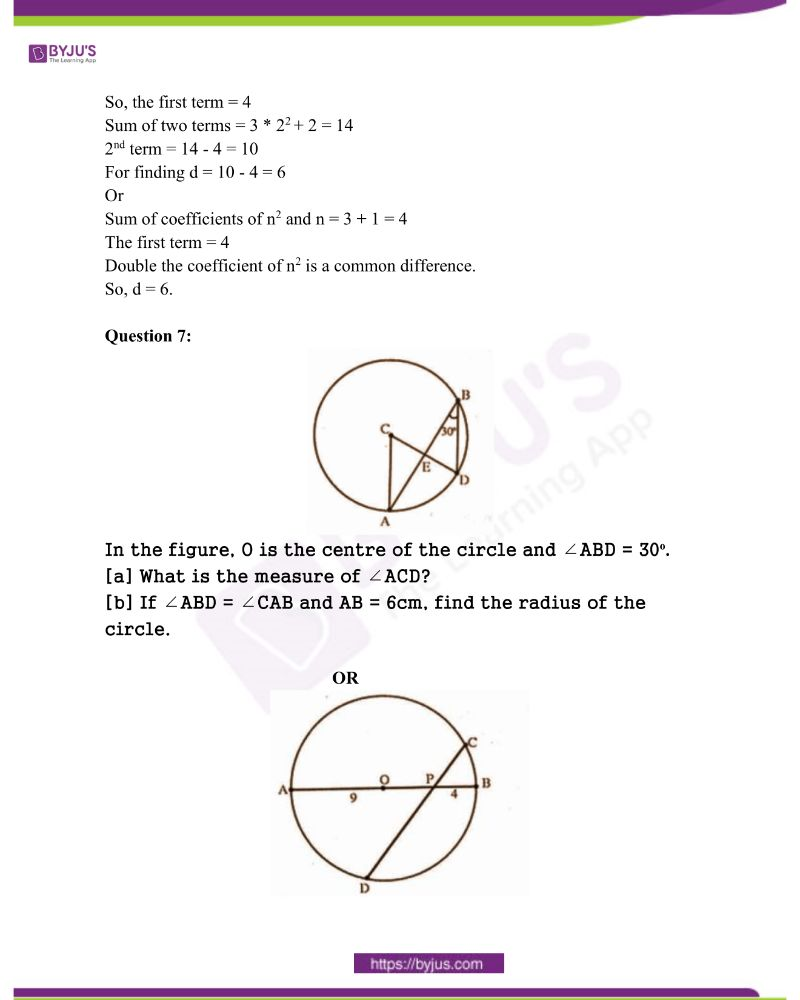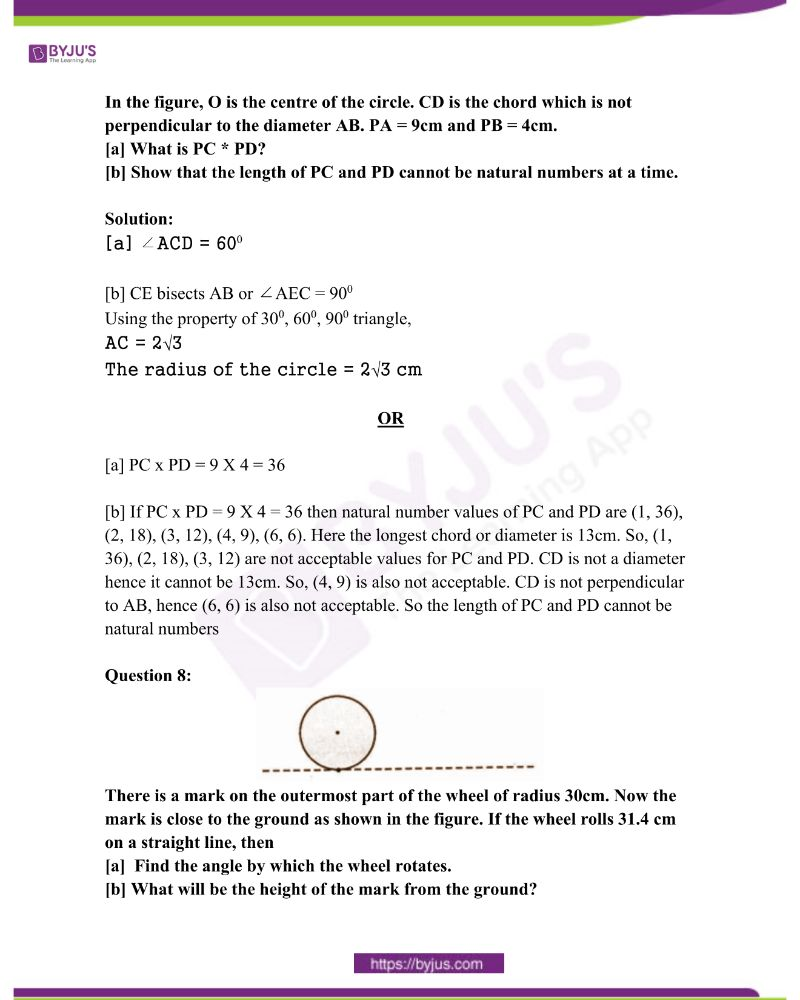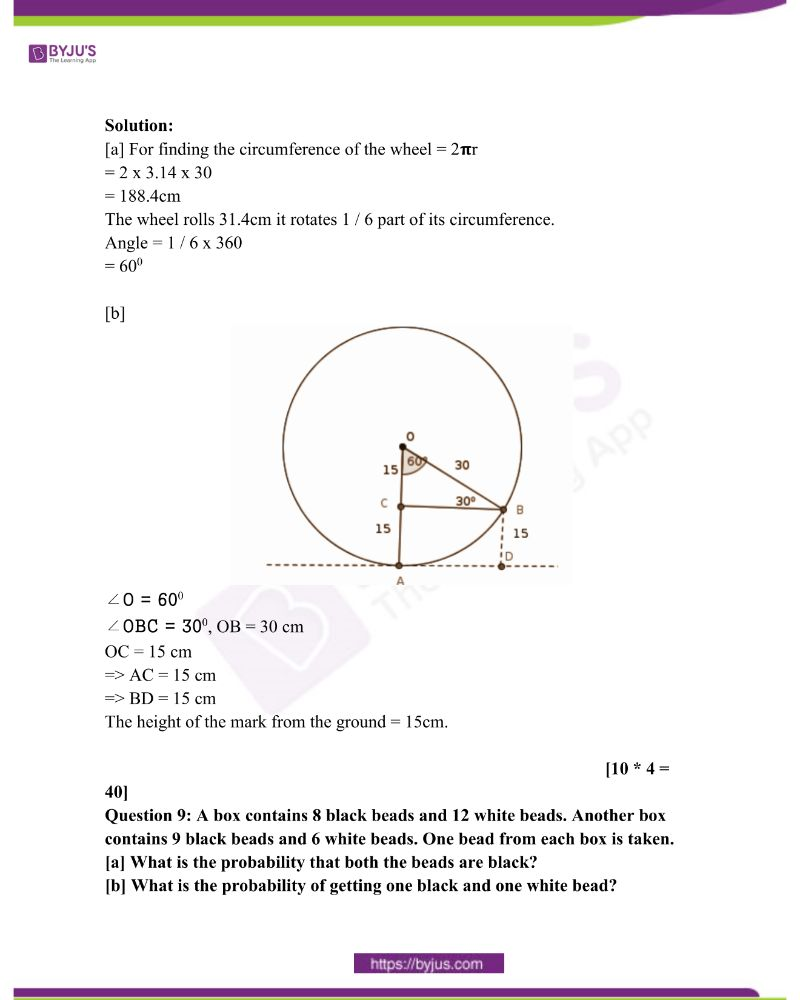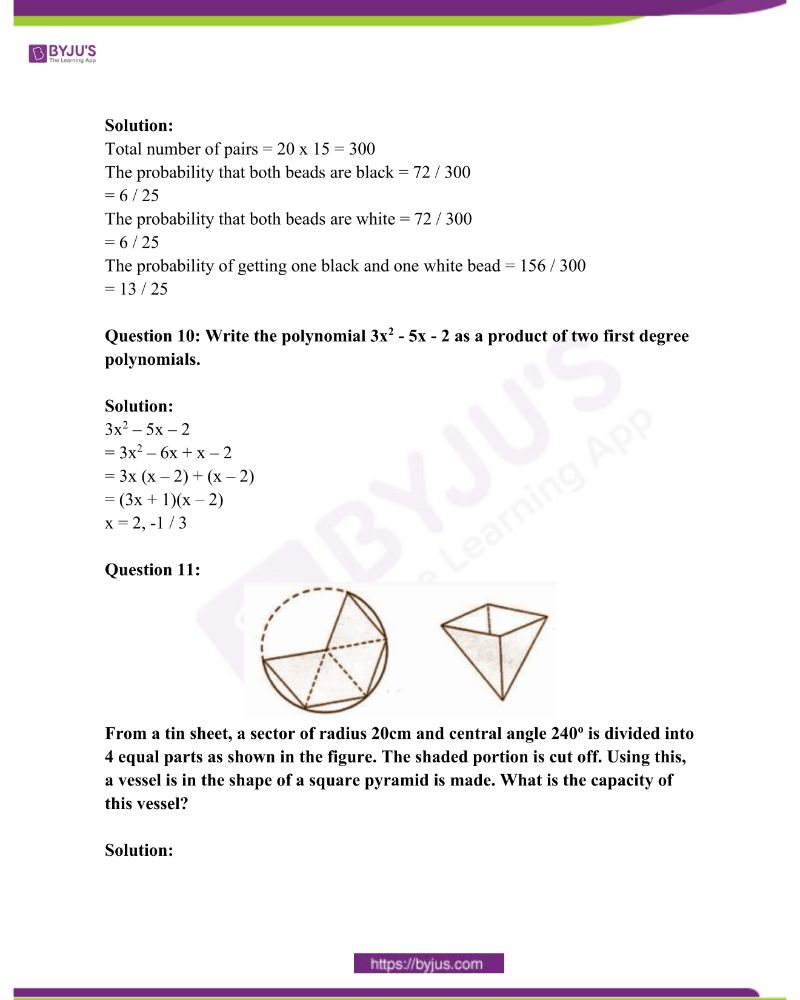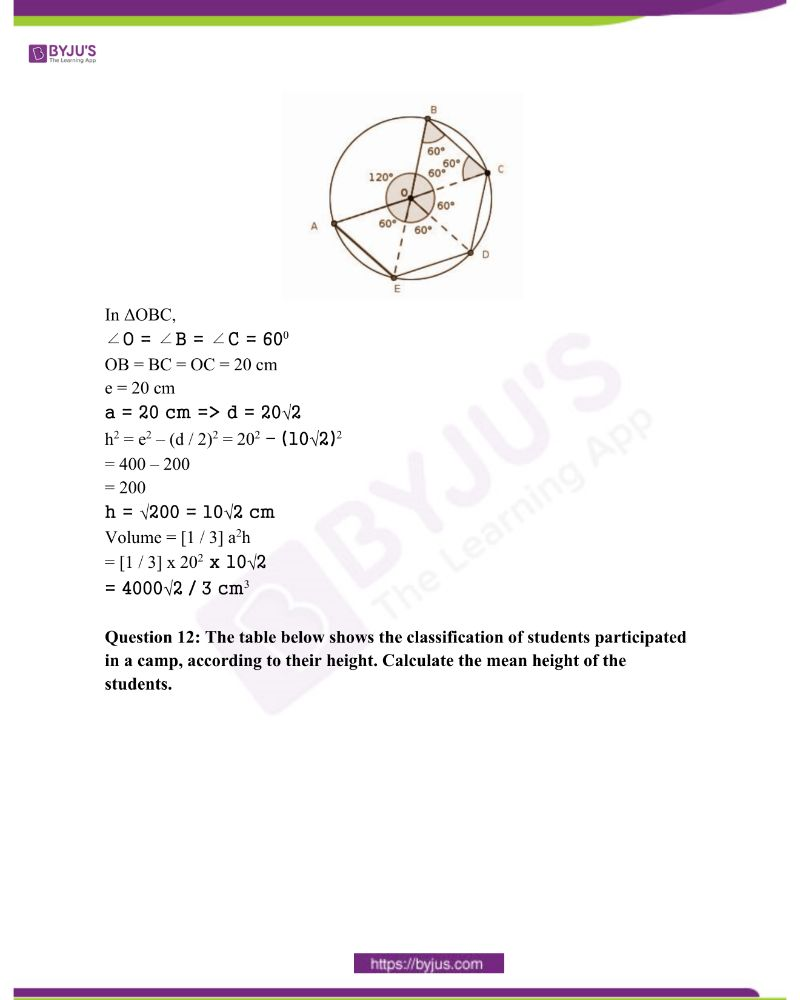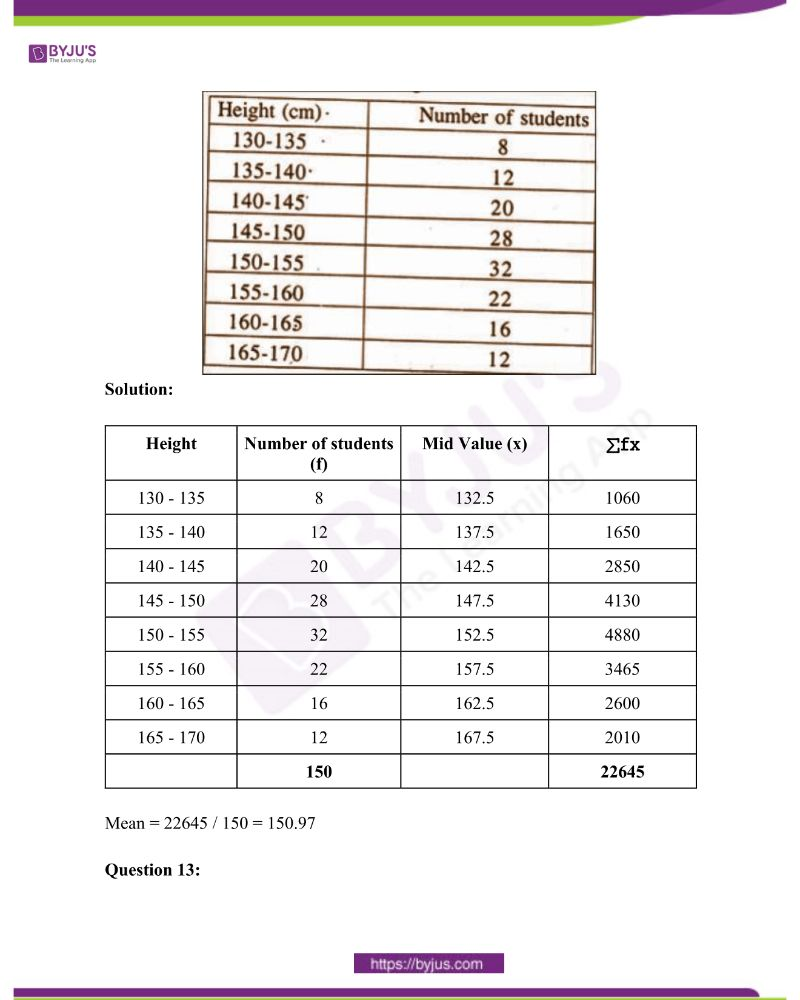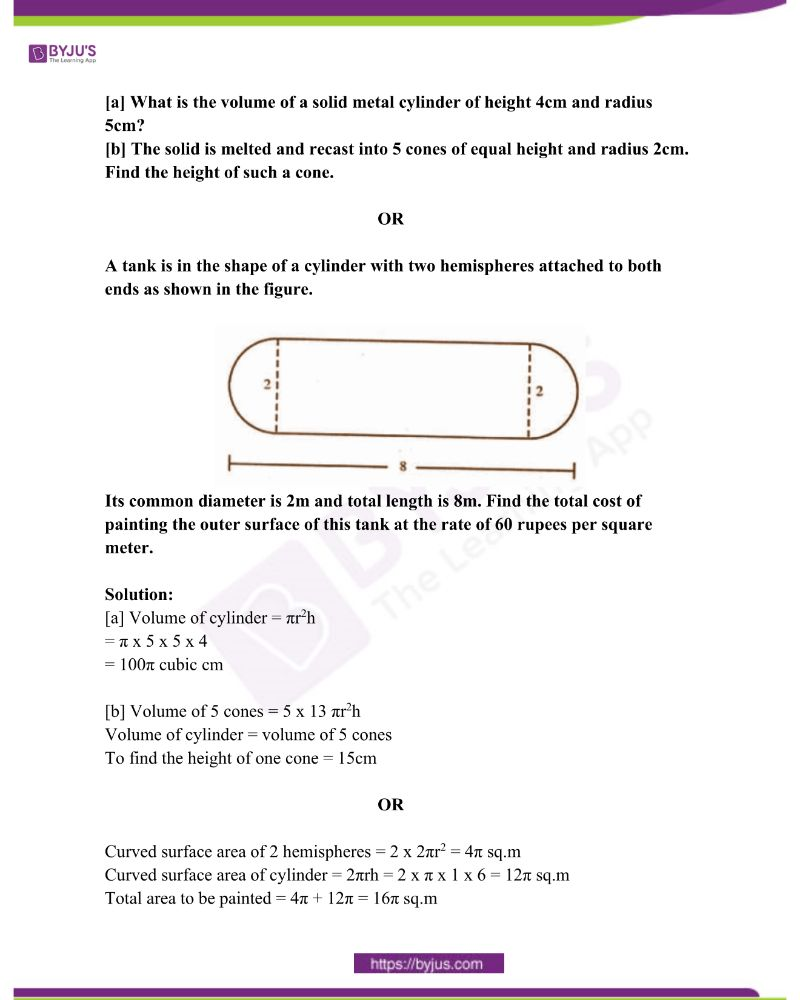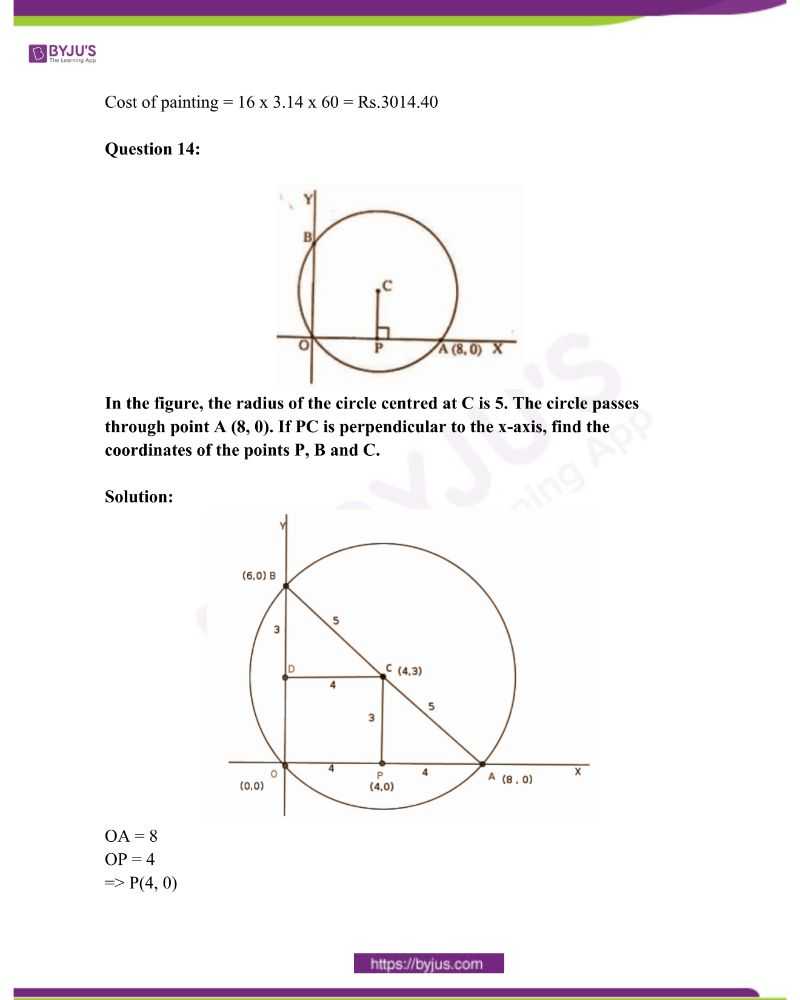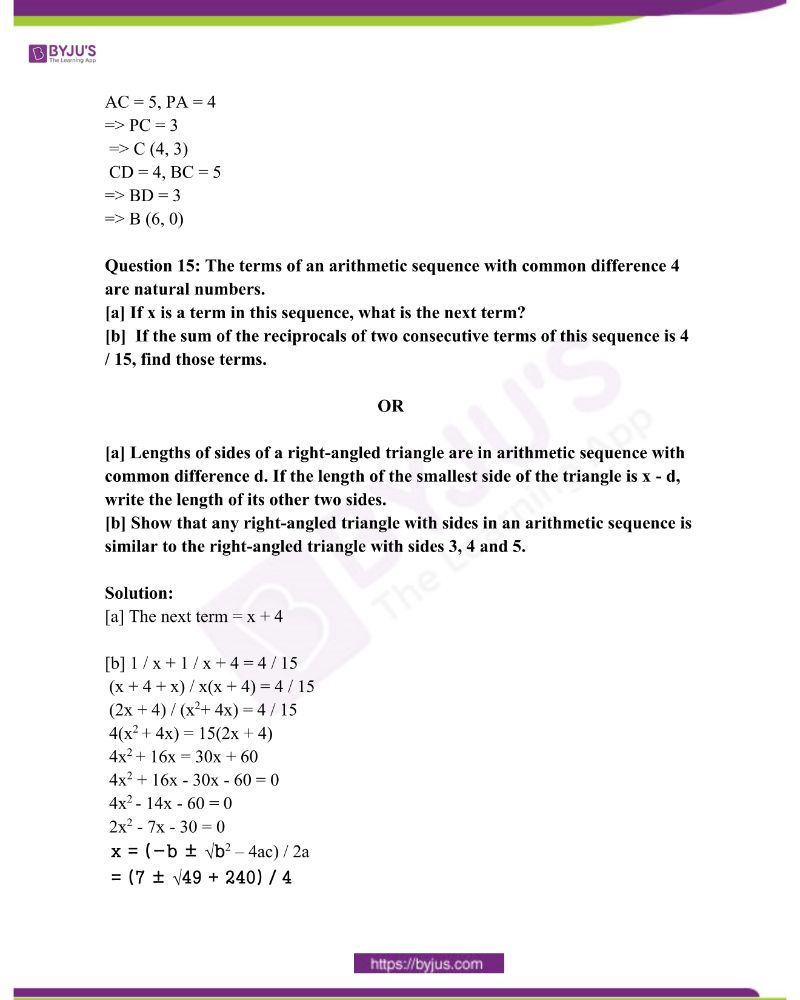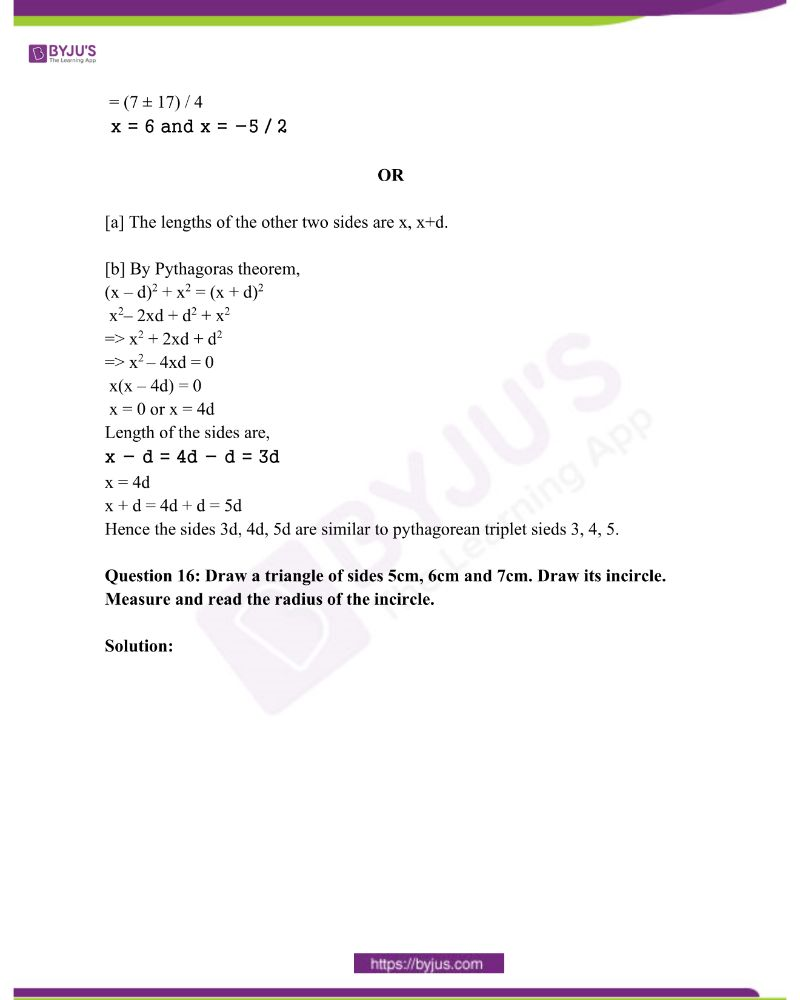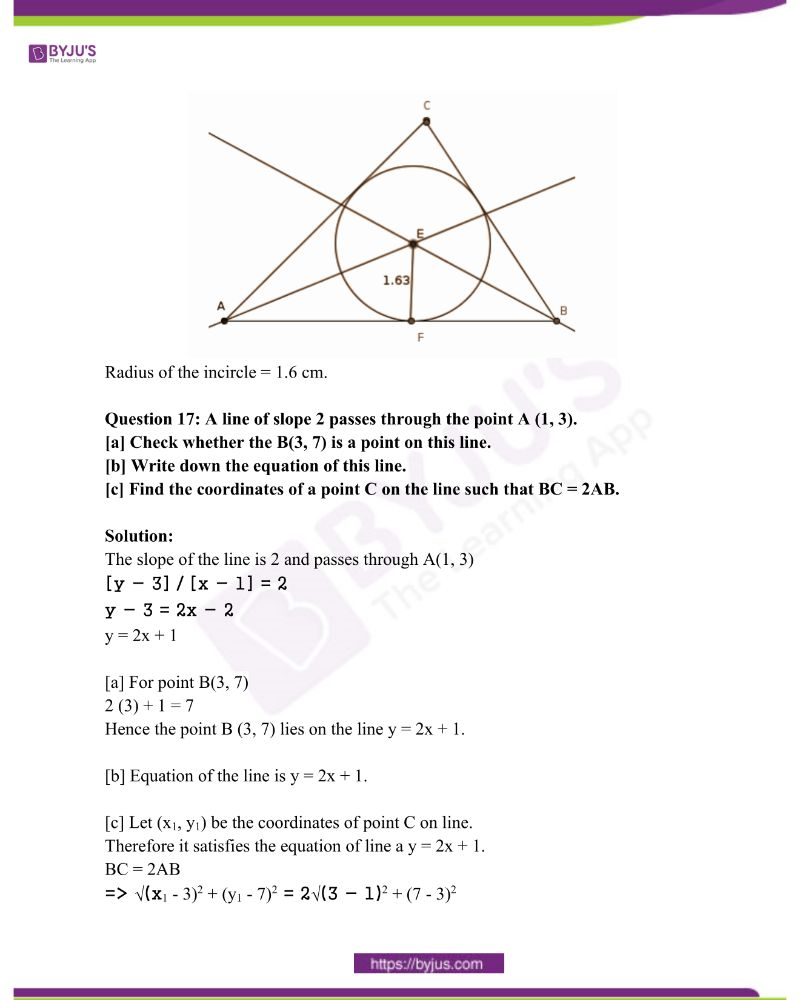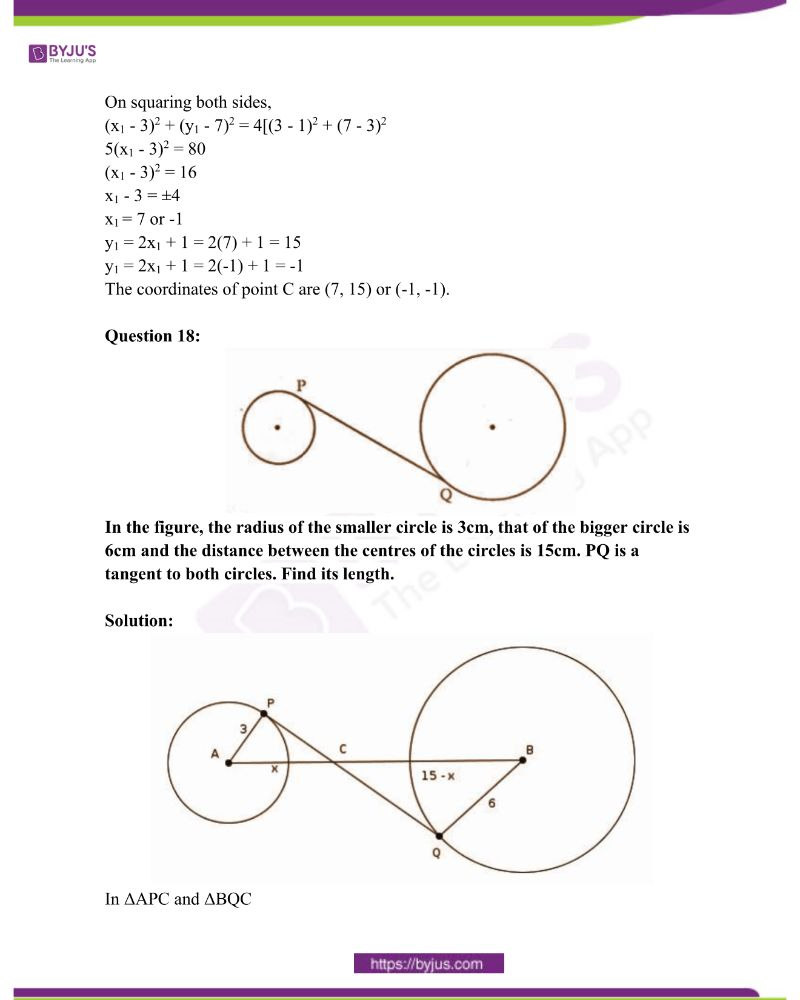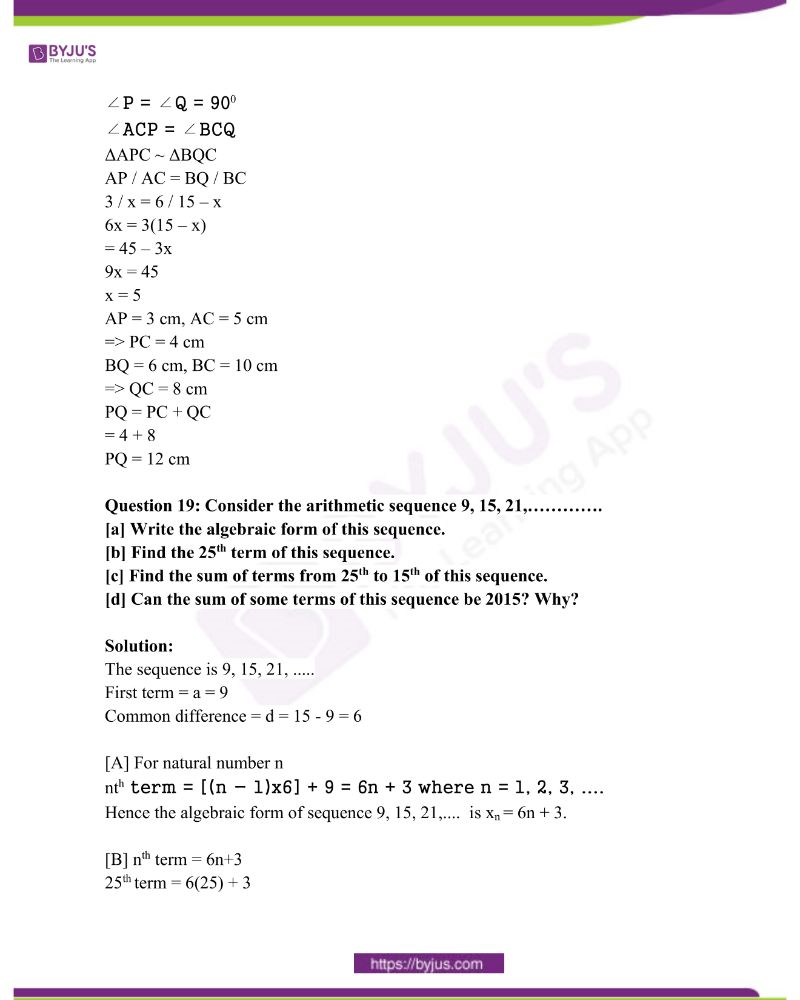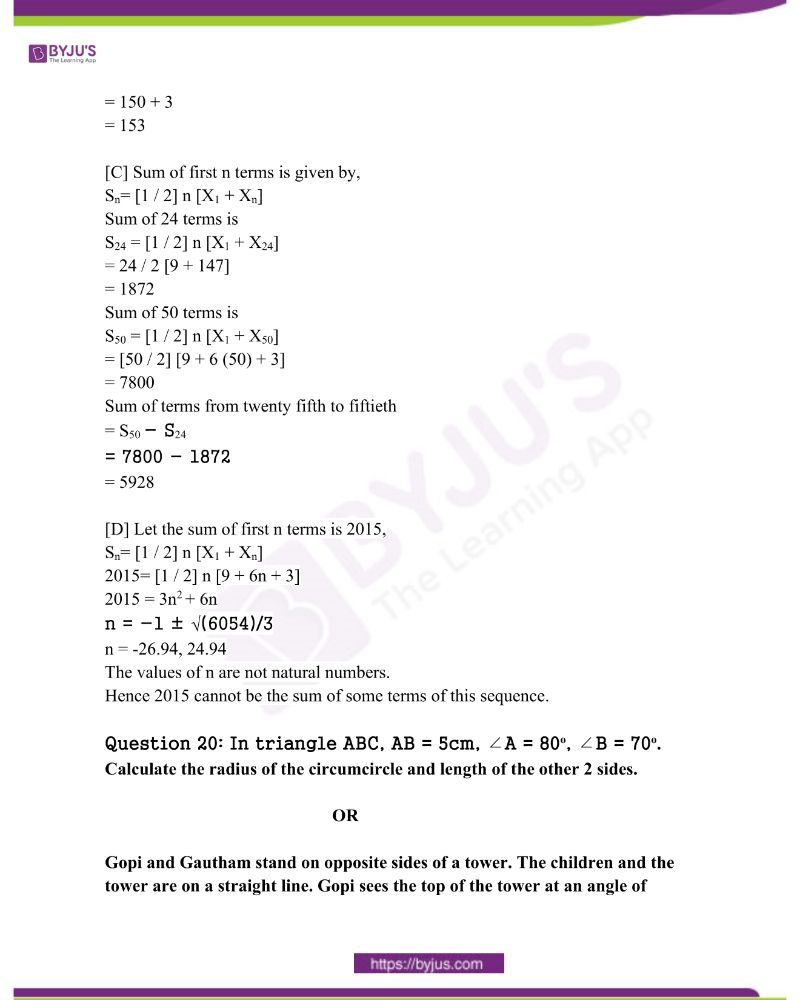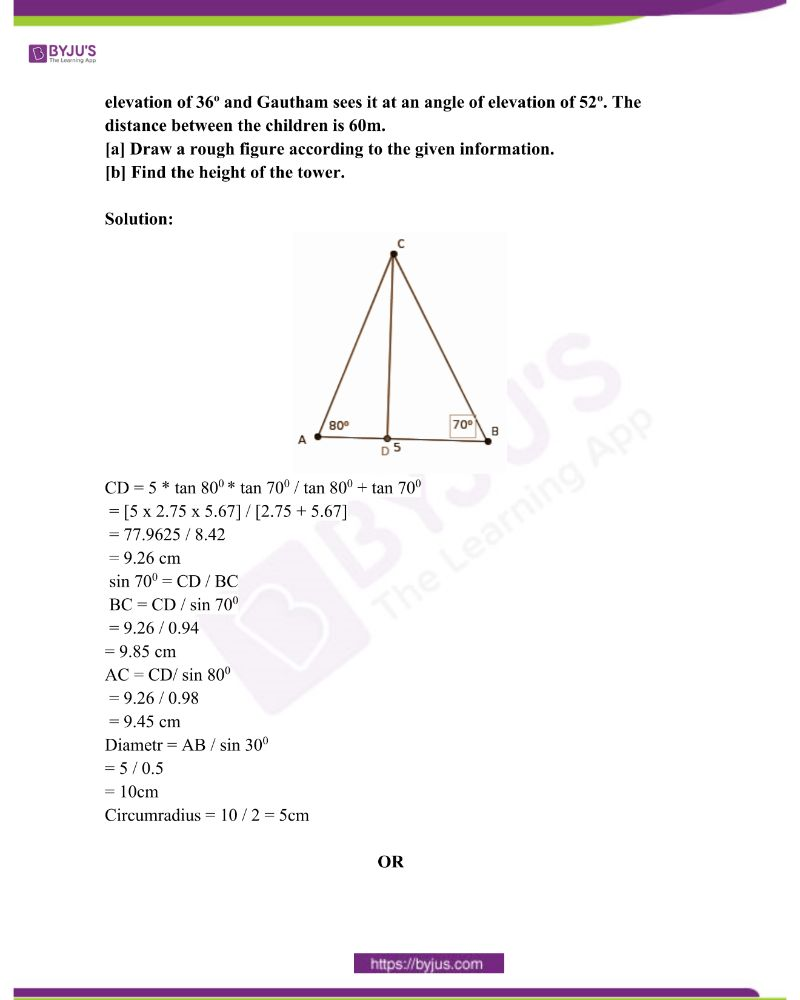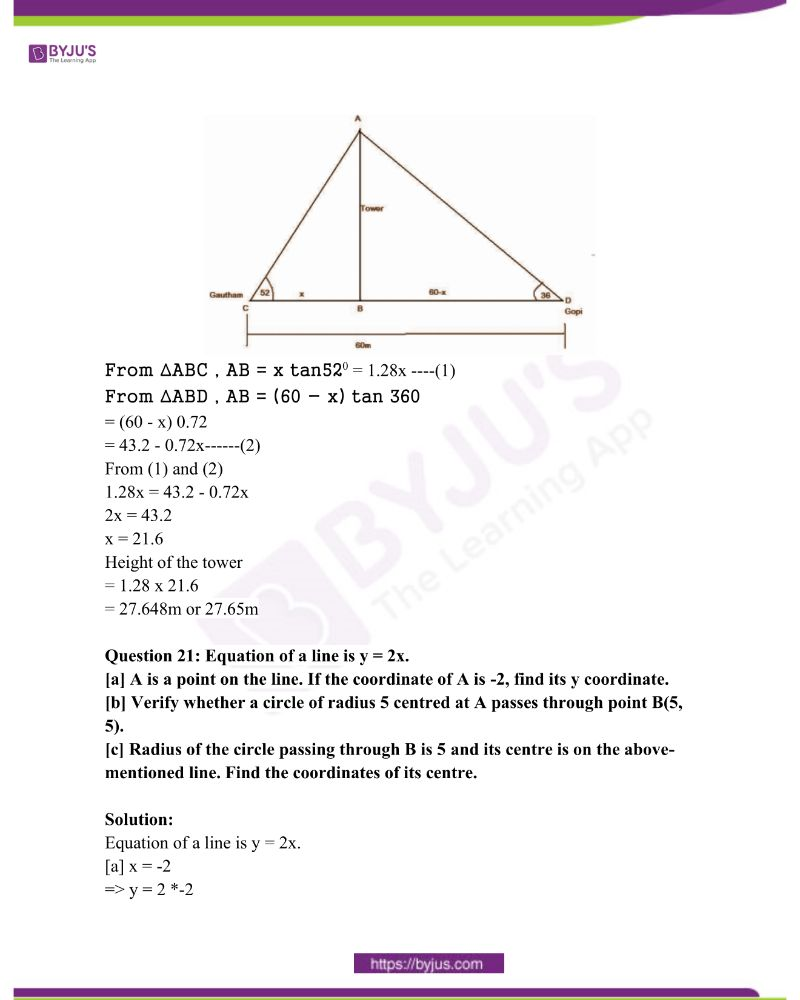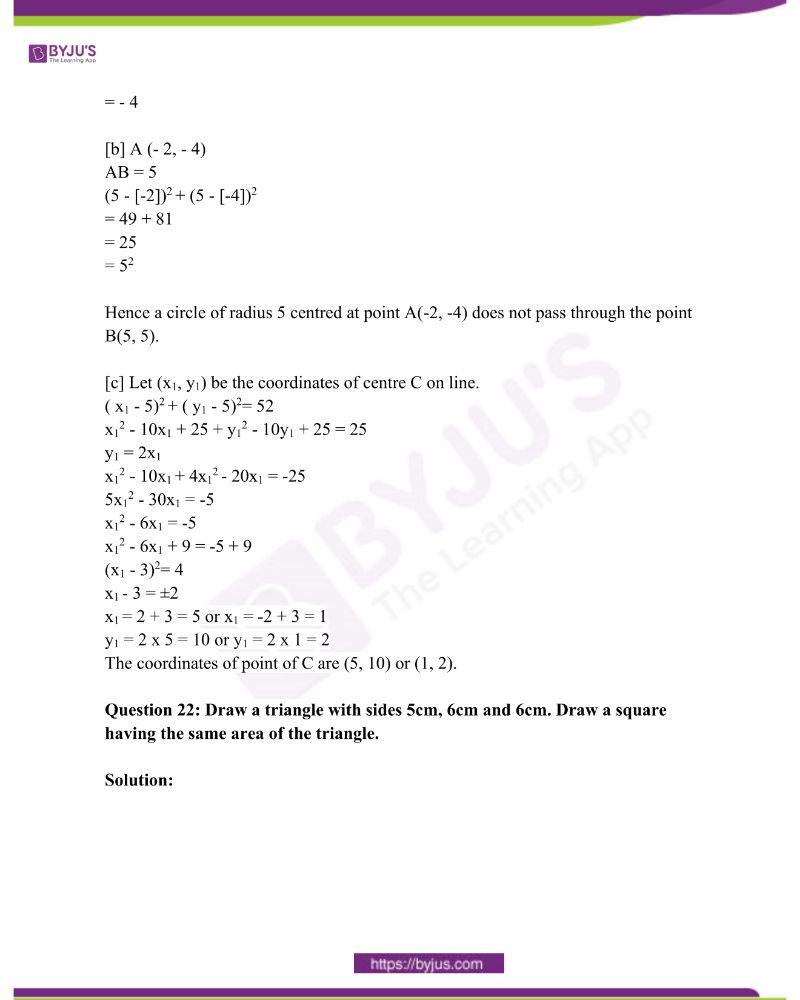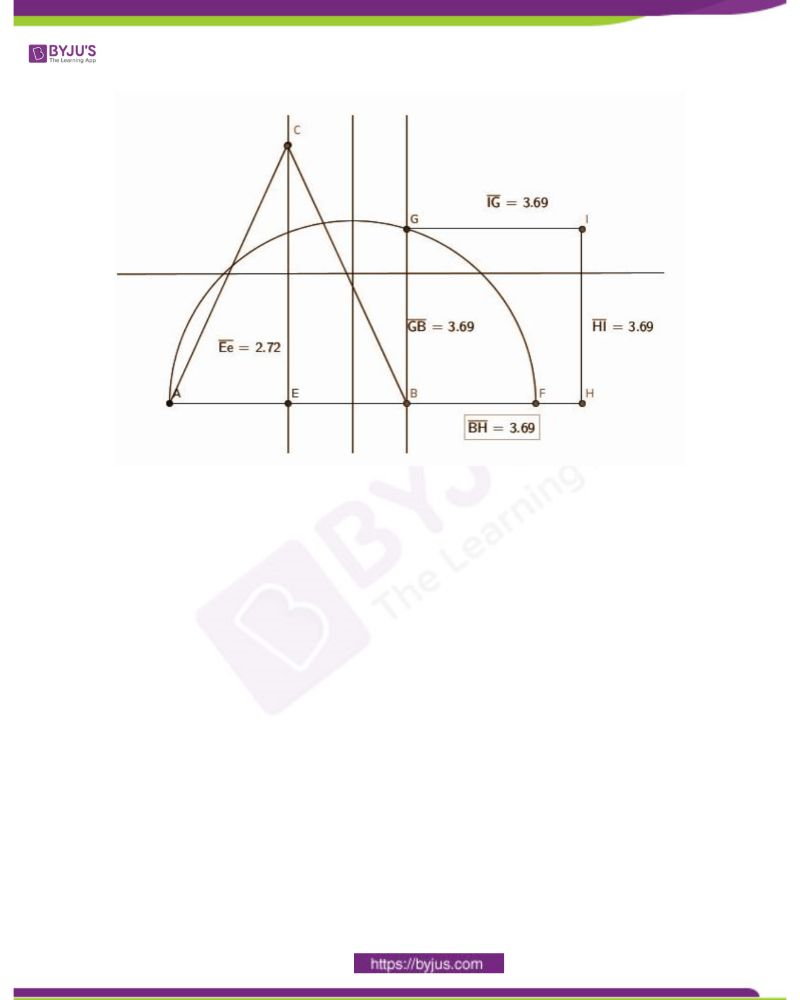Answer the following questions. [2 * 4 = 8]

Question 1: The first term of an arithmetic sequence is 10 and its common difference is 3. Write the first three terms of the sequence. Verify whether 100 is a term of this sequence.

Solution:

The first term of the sequence is 10 and the common difference is 3.

a1= 10 and d = 3

Next term = a2 = a1 + d = 10 + 3 = 13

a3 = a2 + d = 13 + 3 = 16

Thus, the first three terms of the sequence are 10, 13 and 16.

Let 100 be the nth term of the sequence.

an = a1 + (n − 1) d

100=10+(n−1)3

90 = (n − 1) 3

n − 1 = 30

n = 31, which is a whole number.

Therefore, 100 is the 31st term of the sequence.

Question 2: Which number added to the polynomial 3x2 – 4x – 1 gives a polynomial with (x – 1) as a factor?

Solution:

P(1) = 3 (1)2 – 4 (1) – 1 = -2

Add 2 to the given polynomial.

Question 3: If the equation x2 + kx + k = 0 has only one solution, find the possible value of k.

Solution:

x2 + kx + k = 0 has only one solution

The discriminant b2 – 4ac = 0

k2 – 4k = 0

k2 = 4k

k = 0 0r 4

So for k = 0 or k = 4 the equation x2 + 4x + 4 = 0 has only one solution.

Question 4: Draw x and y axes and mark the points A (-1, 2), B (6, 3).

Solution: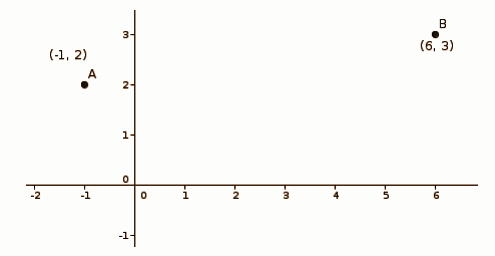[4 * 3 = 12]

Question 5: The scores obtained by 50 students in an examination is tabulated as shown below: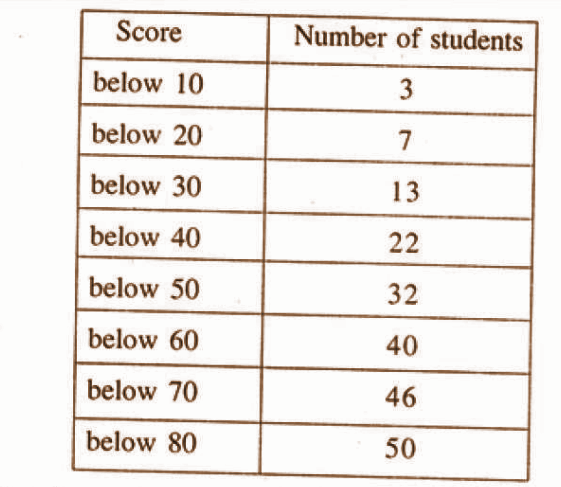Find the median score.

Solution:

 Score 10 20 30 40 50 60 70 80 Number of Students 3 7 13 22 32 40 46 50

y = 50 / 2 = 25

[x – 4] / [50 – 40] = [25 – 22] / [32 – 22]

The median is 43.

Question 6: Sum of first n terms of an arithmetic sequence is 3n2 + n. Find the first term and common difference of this sequence.

Solution:

Sum of 1 term = 3 * 12 + 1 = 4

So, the first term = 4

Sum of two terms = 3 * 22 + 2 = 14

2nd term = 14 – 4 = 10

For finding d = 10 – 4 = 6

Or

Sum of coefficients of n2 and n = 3 + 1 = 4

The first term = 4

Double the coefficient of n2 is a common difference.

So, d = 6.

Question 7: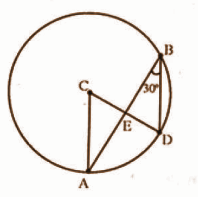In the figure, O is the centre of the circle and ∠ABD = 30o.

[a] What is the measure of ∠ACD?

[b] If ∠ABD = ∠CAB and AB = 6cm, find the radius of the circle.

OR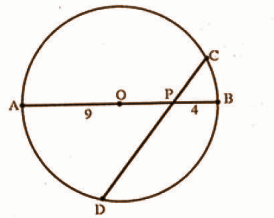In the figure, O is the centre of the circle. CD is the chord which is not perpendicular to the diameter AB. PA = 9cm and PB = 4cm.

[a] What is PC * PD?

[b] Show that the length of PC and PD cannot be natural numbers at a time.

Solution:

[a] ∠ACD = 600

[b] CE bisects AB or AEC = 900

Using the property of 300, 600, 900 triangle,

AC = 2√3

The radius of the circle = 2√3 cm

OR

[a] PC x PD = 9 X 4 = 36

[b] If PC x PD = 9 X 4 = 36 then natural number values of PC and PD are (1, 36), (2, 18), (3, 12), (4, 9), (6, 6). Here the longest chord or diameter is 13cm. So, (1, 36), (2, 18), (3, 12) are not acceptable values for PC and PD. CD is not a diameter hence it cannot be 13cm. So, (4, 9) is also not acceptable. CD is not perpendicular to AB, hence (6, 6) is also not acceptable. So the length of PC and PD cannot be natural numbers

Question 8: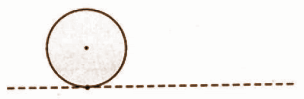There is a mark on the outermost part of the wheel of radius 30cm. Now the mark is close to the ground as shown in the figure. If the wheel rolls 31.4 cm on a straight line, then

[a] Find the angle by which the wheel rotates.

[b] What will be the height of the mark from the ground?

Solution:

[a] For finding the circumference of the wheel = 2𝛑r

= 2 x 3.14 x 30

= 188.4cm

The wheel rolls 31.4cm it rotates 1 / 6 part of its circumference.

Angle = 1 / 6 x 360

= 600

[b]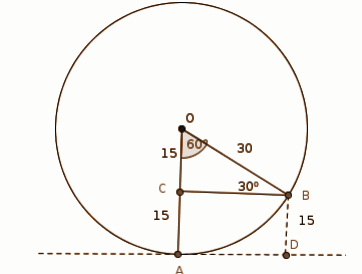∠O = 600

∠OBC = 300, OB = 30 cm

OC = 15 cm

=> AC = 15 cm

=> BD = 15 cm

The height of the mark from the ground = 15cm.

[10 * 4 = 40]

Question 9: A box contains 8 black beads and 12 white beads. Another box contains 9 black beads and 6 white beads. One bead from each box is taken.

[a] What is the probability that both the beads are black?

[b] What is the probability of getting one black and one white bead?

Solution:

Total number of pairs = 20 x 15 = 300

The probability that both beads are black = 72 / 300

= 6 / 25

The probability that both beads are white = 72 / 300

= 6 / 25

The probability of getting one black and one white bead = 156 / 300

= 13 / 25

Question 10: Write the polynomial 3x2 – 5x – 2 as a product of two first degree polynomials.

Solution:

3x2 – 5x – 2

= 3x2 – 6x + x – 2

= 3x (x – 2) + (x – 2)

= (3x + 1)(x – 2)

x = 2, -1 / 3

Question 11: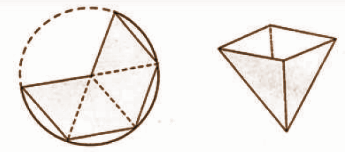From a tin sheet, a sector of radius 20cm and central angle 240o is divided into 4 equal parts as shown in the figure. The shaded portion is cut off. Using this, a vessel in the shape of a square pyramid is made. What is the capacity of this vessel?

Solution: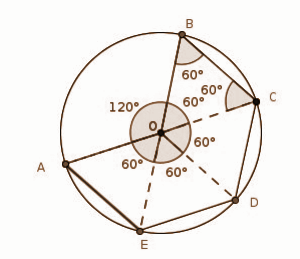In ΔOBC,

∠O = ∠B = ∠C = 600

OB = BC = OC = 20 cm

e = 20 cm

a = 20 cm => d = 20√2

h2 = e2 – (d / 2)2 = 202 – (10√2)2

= 400 – 200

= 200

h = √200 = 10√2 cm

Volume = [1 / 3] a2h

= [1 / 3] x 202 x 10√2

= 4000√2 / 3 cm3

Question 12: The table below shows the classification of students participated in a camp, according to their height. Calculate the mean height of the students.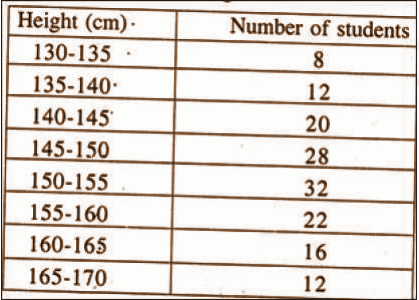Solution:

 Height Number of students (f) Mid Value (x) ∑fx 130 – 135 8 132.5 1060 135 – 140 12 137.5 1650 140 – 145 20 142.5 2850 145 – 150 28 147.5 4130 150 – 155 32 152.5 4880 155 – 160 22 157.5 3465 160 – 165 16 162.5 2600 165 – 170 12 167.5 2010 150 22645

Mean = 22645 / 150 = 150.97

Question 13:

[a] What is the volume of a solid metal cylinder of height 4cm and radius 5cm?

[b] The solid is melted and recast into 5 cones of equal height and radius 2cm. Find the height of such a cone.

OR

A tank is in the shape of a cylinder with two hemispheres attached to both ends as shown in the figure.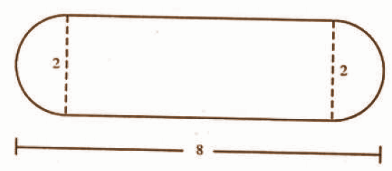Its common diameter is 2m and total length is 8m. Find the total cost of painting the outer surface of this tank at the rate of 60 rupees per square meter.

Solution:

[a] Volume of cylinder = πr2h

= π x 5 x 5 x 4

= 100π cubic cm

[b] Volume of 5 cones = 5 x 13 πr2h

Volume of cylinder = volume of 5 cones

To find the height of one cone = 15cm

OR

Curved surface area of 2 hemispheres = 2 x 2πr2 = 4π sq.m

Curved surface area of cylinder = 2πrh = 2 x π x 1 x 6 = 12π sq.m

Total area to be painted = 4π + 12π = 16π sq.m

Cost of painting = 16 x 3.14 x 60 = Rs.3014.40

Question 14: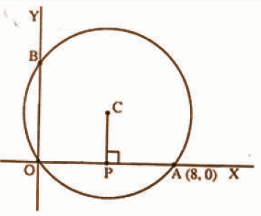In the figure, the radius of the circle centred at C is 5. The circle passes through point A (8, 0). If PC is perpendicular to the x-axis, find the coordinates of the points P, B and C.

Solution: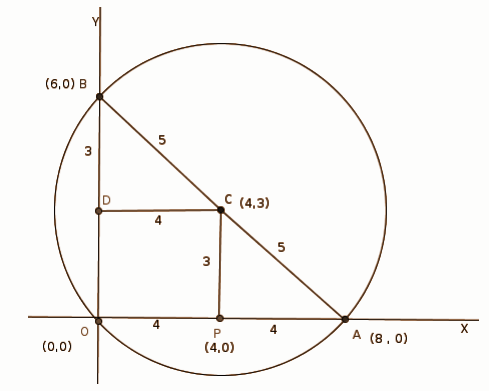OA = 8

OP = 4

=> P(4, 0)

AC = 5, PA = 4

=> PC = 3

=> C (4, 3)

CD = 4, BC = 5

=> BD = 3

=> B (6, 0)

Question 15: The terms of an arithmetic sequence with common difference 4 are natural numbers.

[a] If x is a term in this sequence, what is the next term?

[b] If the sum of the reciprocals of two consecutive terms of this sequence is 4 / 15, find those terms.

OR

[a] Lengths of sides of a right-angled triangle are in arithmetic sequence with common difference d. If the length of the smallest side of the triangle is x – d, write the length of its other two sides.

[b] Show that any right-angled triangle with sides in an arithmetic sequence is similar to the right-angled triangle with sides 3, 4 and 5.

Solution:

[a] The next term = x + 4

[b] 1 / x + 1 / x + 4 = 4 / 15

(x + 4 + x) / x(x + 4) = 4 / 15

(2x + 4) / (x2+ 4x) = 4 / 15

4(x2 + 4x) = 15(2x + 4)

4x2 + 16x = 30x + 60

4x2 + 16x – 30x – 60 = 0

4x2 – 14x – 60 = 0

2x2 – 7x – 30 = 0

x = (-b ± √b2 – 4ac) / 2a

= (7 ± √49 + 240) / 4

= (7 ± 17) / 4

x = 6 and x = −5 / 2

OR

[a] The lengths of the other two sides are x, x+d.

[b] By Pythagoras theorem,

(x – d)2 + x2 = (x + d)2

x2– 2xd + d2 + x2

=> x2 + 2xd + d2

=> x2 – 4xd = 0

x(x – 4d) = 0

x = 0 or x = 4d

Length of the sides are,

x − d = 4d − d = 3d

x = 4d

x + d = 4d + d = 5d

Hence the sides 3d, 4d, 5d are similar to pythagorean triplet sieds 3, 4, 5.

Question 16: Draw a triangle of sides 5cm, 6cm and 7cm. Draw its incircle. Measure and read the radius of the incircle.

Solution: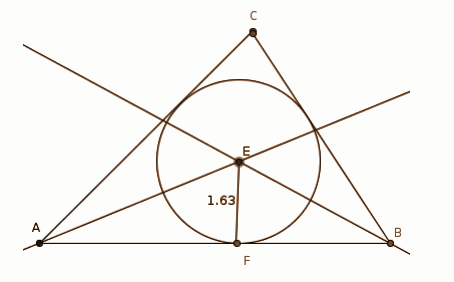Radius of the incircle = 1.6 cm.

Question 17: A line of slope 2 passes through the point A (1, 3).

[a] Check whether the B(3, 7) is a point on this line.

[b] Write down the equation of this line.

[c] Find the coordinates of a point C on the line such that BC = 2AB.

Solution:

The slope of the line is 2 and passes through A(1, 3)

[y − 3] / [x − 1] = 2

y − 3 = 2x − 2

y = 2x + 1

[a] For point B(3, 7)

2 (3) + 1 = 7

Hence the point B (3, 7) lies on the line y = 2x + 1.

[b] Equation of the line is y = 2x + 1.

[c] Let (x1, y1) be the coordinates of point C on line.

Therefore it satisfies the equation of line a y = 2x + 1.

BC = 2AB

=> √(x1 – 3)2 + (y1 – 7)2 = 2√(3 – 1)2 + (7 – 3)2

On squaring both sides,

(x1 – 3)2 + (y1 – 7)2 = 4[(3 – 1)2 + (7 – 3)2

5(x1 – 3)2 = 80

(x1 – 3)2 = 16

x1 – 3 = ±4

x1 = 7 or -1

y1 = 2x1 + 1 = 2(7) + 1 = 15

y1 = 2x1 + 1 = 2(-1) + 1 = -1

The coordinates of point C are (7, 15) or (-1, -1).

Question 18: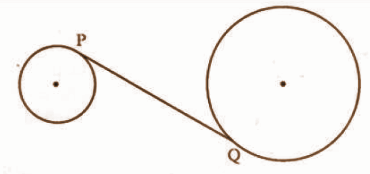In the figure, the radius of the smaller circle is 3cm, that of the bigger circle is 6cm and the distance between the centres of the circles is 15cm. PQ is a tangent to both circles. Find its length.

Solution: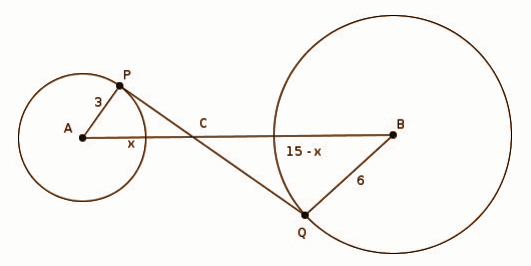In ΔAPC and ΔBQC

∠P = ∠Q = 900

∠ACP = ∠BCQ

ΔAPC ~ ΔBQC

AP / AC = BQ / BC

3 / x = 6 / 15 – x

6x = 3(15 – x)

= 45 – 3x

9x = 45

x = 5

AP = 3 cm, AC = 5 cm

=> PC = 4 cm

BQ = 6 cm, BC = 10 cm

=> QC = 8 cm

PQ = PC + QC

= 4 + 8

PQ = 12 cm

Question 19: Consider the arithmetic sequence 9, 15, 21,………….

[a] Write the algebraic form of this sequence.

[b] Find the 25th term of this sequence.

[c] Find the sum of terms from 25th to 15th of this sequence.

[d] Can the sum of some terms of this sequence be 2015? Why?

Solution:

The sequence is 9, 15, 21, …..

First term = a = 9

Common difference = d = 15 – 9 = 6

[A] For natural number n

nth term = [(n − 1)x6] + 9 = 6n + 3 where n = 1, 2, 3, ….

Hence the algebraic form of sequence 9, 15, 21,…. is xn = 6n + 3.

[B] nth term = 6n+3

25th term = 6(25) + 3

= 150 + 3

= 153

[C] Sum of first n terms is given by,

Sn= [1 / 2] n [X1 + Xn]

Sum of 24 terms is

S24 = [1 / 2] n [X1 + X24]

= 24 / 2 [9 + 147]

= 1872

Sum of 50 terms is

S50 = [1 / 2] n [X1 + X50]

= [50 / 2] [9 + 6 (50) + 3]

= 7800

Sum of terms from twenty fifth to fiftieth

= S50 − S24

= 7800 − 1872

= 5928

[D] Let the sum of first n terms is 2015,

Sn= [1 / 2] n [X1 + Xn]

2015= [1 / 2] n [9 + 6n + 3]

2015 = 3n2 + 6n

n = -1 ± √(6054)/3

n = -26.94, 24.94

The values of n are not natural numbers.

Hence 2015 cannot be the sum of some terms of this sequence.

Question 20: In triangle ABC, AB = 5cm, ∠A = 80o, ∠B = 70o. Calculate the radius of the circumcircle and length of the other 2 sides.

OR

Gopi and Gautham stand on opposite sides of a tower. The children and the tower are on a straight line. Gopi sees the top of the tower at an angle of elevation of 36o and Gautham sees it at an angle of elevation of 52o. The distance between the children is 60m.

[a] Draw a rough figure according to the given information.

[b] Find the height of the tower.

Solution: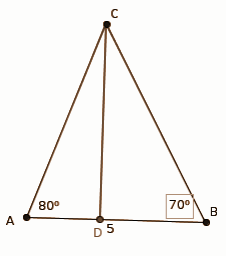CD = 5 * tan 800 * tan 700 / tan 800 + tan 700

= [5 x 2.75 x 5.67] / [2.75 + 5.67]

= 77.9625 / 8.42

= 9.26 cm

sin 700 = CD / BC

BC = CD / sin 700

= 9.26 / 0.94

= 9.85 cm

AC = CD/ sin 800

= 9.26 / 0.98

= 9.45 cm

Diameter = AB / sin 300

= 5 / 0.5

= 10cm

Circumradius = 10 / 2 = 5cm

OR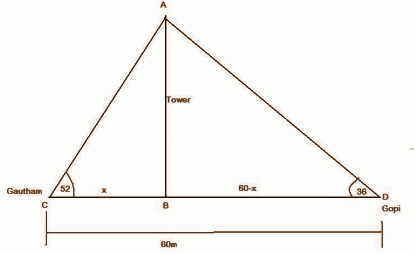From ∆ABC , AB = x tan520 = 1.28x —-(1)

From ∆ABD , AB = (60 – x) tan 360

= (60 – x) 0.72

= 43.2 – 0.72x——(2)

From (1) and (2)

1.28x = 43.2 – 0.72x

2x = 43.2

x = 21.6

Height of the tower

= 1.28 x 21.6

= 27.648m or 27.65m

Question 21: Equation of a line is y = 2x.

[a] A is a point on the line. If the coordinate of A is -2, find its y coordinate.

[b] Verify whether a circle of radius 5 centred at A passes through point B(5, 5).

[c] Radius of the circle passing through B is 5 and its centre is on the above-mentioned line. Find the coordinates of its centre.

Solution:

Equation of a line is y = 2x.

[a] x = -2

=> y = 2 *-2

= – 4

[b] A (- 2, – 4)

AB = 5

(5 – [-2])2 + (5 – [-4])2

= 49 + 81

= 25

= 52

Hence a circle of radius 5 centred at point A(-2, -4) does not pass through the point B(5, 5).

[c] Let (x1, y1) be the coordinates of centre C on line.

( x1 – 5)2 + ( y1 – 5)2= 52

x12 – 10x1 + 25 + y12 – 10y1 + 25 = 25

y1 = 2x1

x12 – 10x1 + 4x12 – 20x1 = -25

5x12 – 30x1 = -5

x12 – 6x1 = -5

x12 – 6x1 + 9 = -5 + 9

(x1 – 3)2= 4

x1 – 3 = ±2

x1 = 2 + 3 = 5 or x1 = -2 + 3 = 1

y1 = 2 x 5 = 10 or y1 = 2 x 1 = 2

The coordinates of point of C are (5, 10) or (1, 2).

Question 22: Draw a triangle with sides 5cm, 6cm and 6cm. Draw a square having the same area of the triangle.

Solution: